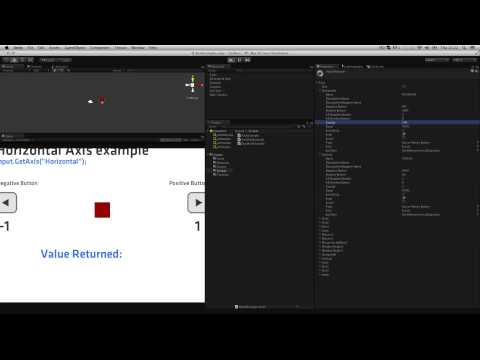# GetAxis

#### Difficulty: Beginner

How to "get axis" based input for your games in Unity and how these axes can be modified with the Input manager## GetAxis

Beginner Scripting

### Code snippet

``````using UnityEngine;
using System.Collections;

public class AxisExample : MonoBehaviour
{
public float range;
public GUIText textOutput;

void Update ()
{
float h = Input.GetAxis("Horizontal");
float xPos = h * range;

transform.position = new Vector3(xPos, 2f, 0);
textOutput.text = "Value Returned: "+h.ToString("F2");
}
}``````
``````#pragma strict

public var range : float;
public var textOutput : GUIText;

function Update ()
{
var h : float = Input.GetAxis("Horizontal");
var xPos : float = h * range;

transform.position = new Vector3(xPos, 2f, 0);
textOutput.text = "Value Returned: "+h.ToString("F2");
}``````
``````import UnityEngine
import System.Collections

public class AxisExample(MonoBehaviour):

public range as single

public textOutput as GUIText

private def Update():
h as single = Input.GetAxis('Horizontal')
xPos as single = (h * range)

transform.position = Vector3(xPos, 2.0F, 0)
textOutput.text = ('Value Returned: ' + h.ToString('F2'))``````

### Code snippet

``````using UnityEngine;
using System.Collections;

public class AxisRawExample : MonoBehaviour
{
public float range;
public GUIText textOutput;

void Update ()
{
float h = Input.GetAxisRaw("Horizontal");
float xPos = h * range;

transform.position = new Vector3(xPos, 2f, 0);
textOutput.text = "Value Returned: "+h.ToString("F2");
}
}``````
``````#pragma strict

public var range : float;
public var textOutput : GUIText;

function Update ()
{
var h : float = Input.GetAxisRaw("Horizontal");
var xPos : float = h * range;

transform.position = new Vector3(xPos, 2f, 0);
textOutput.text = "Value Returned: "+h.ToString("F2");
}``````
``````import UnityEngine
import System.Collections

public class AxisRawExample(MonoBehaviour):

public range as single

public textOutput as GUIText

private def Update():
h as single = Input.GetAxisRaw('Horizontal')
xPos as single = (h * range)

transform.position = Vector3(xPos, 2.0F, 0)
textOutput.text = ('Value Returned: ' + h.ToString('F2'))``````

### Code snippet

``````using UnityEngine;
using System.Collections;

public class DualAxisExample : MonoBehaviour
{
public float range;
public GUIText textOutput;

void Update ()
{
float h = Input.GetAxis("Horizontal");
float v = Input.GetAxis("Vertical");
float xPos = h * range;
float yPos = v * range;

transform.position = new Vector3(xPos, yPos, 0);
textOutput.text = "Horizontal Value Returned: "+h.ToString("F2")+"\nVertical Value Returned: "+v.ToString("F2");
}
}``````
``````#pragma strict

public var range : float;
public var textOutput : GUIText;

function Update ()
{
var h : float = Input.GetAxis("Horizontal");
var v : float = Input.GetAxis("Vertical");
var xPos : float = h * range;
var yPos : float = v * range;

transform.position = new Vector3(xPos, yPos, 0);
textOutput.text = "Horizontal Value Returned: "+h.ToString("F2")+"\nVertical Value Returned: "+v.ToString("F2");
}``````
``````import UnityEngine
import System.Collections

public class DualAxisExample(MonoBehaviour):

public range as single

public textOutput as GUIText

private def Update():
h as single = Input.GetAxis('Horizontal')
v as single = Input.GetAxis('Vertical')
xPos as single = (h * range)
yPos as single = (v * range)

transform.position = Vector3(xPos, yPos, 0)
textOutput.text = ((('Horizontal Value Returned: ' + h.ToString('F2')) + '\nVertical Value Returned: ') + v.ToString('F2'))``````

#### Related documentation